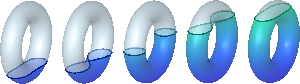# Vaughn Climenhaga

## Associate ProfessorDepartment of MathematicsUniversity of HoustonI am teaching one course in Fall 2021: F'21 - Math 2413 - Calculus I (invitation-only section) Courses I taught in previous terms are listed below. S'21 - Math 4362 - Theory of Differential Equations and Nonlinear Dynamics F'20 - Math 6342 - Topology F'20 - Math 3331 - Differential equations S'20 - Math 1432 - Calculus II (invitation-only section) F'19 - Math 1431 - Calculus I (invitation-only section) F'19 - Math 7326 - Dynamical Systems S'19 - Math 1432 - Calculus II (invitation-only section) S'19 - Math 7394 - Ergodic theory & thermodynamic formalism F'18 - Math 1431 - Calculus I (invitation-only section) S'18 - Math 6321 - Functions of a real variable II S'18 - Math 7352 - Riemannian geometry S'17 - Math 7326 - Dynamical Systems S'17 - Math 1432 - Calculus II (invitation-only section) F'16 - Math 1431 - Calculus I (invitation-only section) S'16 - Math 6321 - Functions of a real variable II F'15 - Math 3338 - Probability F'15 - Math 6320 - Functions of a real variable I S'15 - Math 7350 - Geometry of Manifolds F'14 - Math 6342 - Topology S'14 - Math 7350 - Geometry of Manifolds F'13 - Math 4377/6308 - Advanced Linear Algebra I S'13 - Math 4377/6308 - Advanced Linear Algebra I (link to course notes at right) F'12 - Math 6324 - Ordinary Differential Equations S'12 - Math 224 (University of Toronto) - Linear Algebra 2 F'10 - Math 112 (University of Maryland) - Algebra and Trigonometry S'09 - Math 230 (Pennsylvania State University) - Calculus and Vector Analysis Sum'06 - Math 35 (PSU) - Survey of Mathematical Thought S'2006 - Math 26 (PSU) - Plane TrigonometryCourse notes for Math 4377, Advanced Linear Algebra I

Houston Workshop on Hyperbolic Dynamical Systems

Pages for previous summer schools: